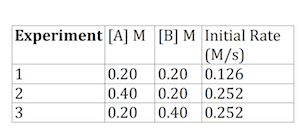# Determining Rate Equation, Rate Law Constant & Reaction Order from Experimental Data - Quiz & Worksheet

Instructions:

Choose an answer and hit 'next'. You will receive your score and answers at the end.

question 1 of 3

### Based on experimental data, you learned that x = 2 and y = 1 in a rate law equation. What will the reaction order be?

Create Your Account To Take This Quiz

As a member, you'll also get unlimited access to over 84,000 lessons in math, English, science, history, and more. Plus, get practice tests, quizzes, and personalized coaching to help you succeed.

Try it risk-free for 30 days. Cancel anytime

### 1. Which of the following is TRUE about the following data with regard to determining x and y in a rate law?### 2. If x = 1 and y = 1, use the data to determine k, or the rate law.Create your account to access this entire worksheet
A Premium account gives you access to all lesson, practice exams, quizzes & worksheets
Quizzes, practice exams & worksheets
Certificate of Completion
Create an account to get started

You can test your knowledge of the process of finding rate equation, law constant, and reaction order from experimental data with this quiz and worksheet. For example, you should know how to determine x and y in a rate law.

## Quiz & Worksheet Goals

You'll be quizzed on the following:

• Finding the reaction order
• A true statement about determining x and y in a rate law
• Using specified data to determine the rate law
• A true statement about the rate law
• Identifying a variable that's assigned to a rate constant

## Skills Practiced

• Interpreting information - verify that you can read information about determining the rate law and interpret it correctly
• Problem solving - use acquired knowledge to solve reaction order problems
• Information recall - access the knowledge you've gained about determining a rate constant

To take a closer look at this topic, review the lesson titled Determining Rate Equation, Rate Law Constant & Reaction Order from Experimental Data. Here are a few examples of what's included in this lesson:

• A rate law equation
• How the reaction order is used
• A reaction with the experimental data
Final ExamHigh School Chemistry: Help and Review
Status: Not Started
Chapter ExamChemical Reactions, Rate Laws & Constants
Status: Not Started

Support